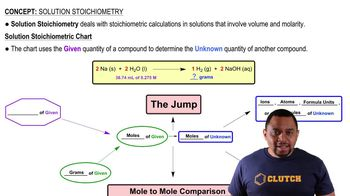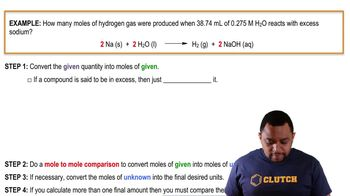Start typing, then use the up and down arrows to select an option from the list.
7:30 minutes
Problem 142
Textbook Question

# Morphine has the formula C17H19NO3. It is a base and accepts one proton per molecule. It is isolated from opium. A 0.682-g sample of opium is found to require 8.92 mL of a 0.0116 M solution of sulfuric acid for neutralization. Assuming that morphine is the only acid or base present in opium, calculate the percent morphine in the sample of opium.Verified Solution
This video solution was recommended by our tutors as helpful for the problem above.
571views